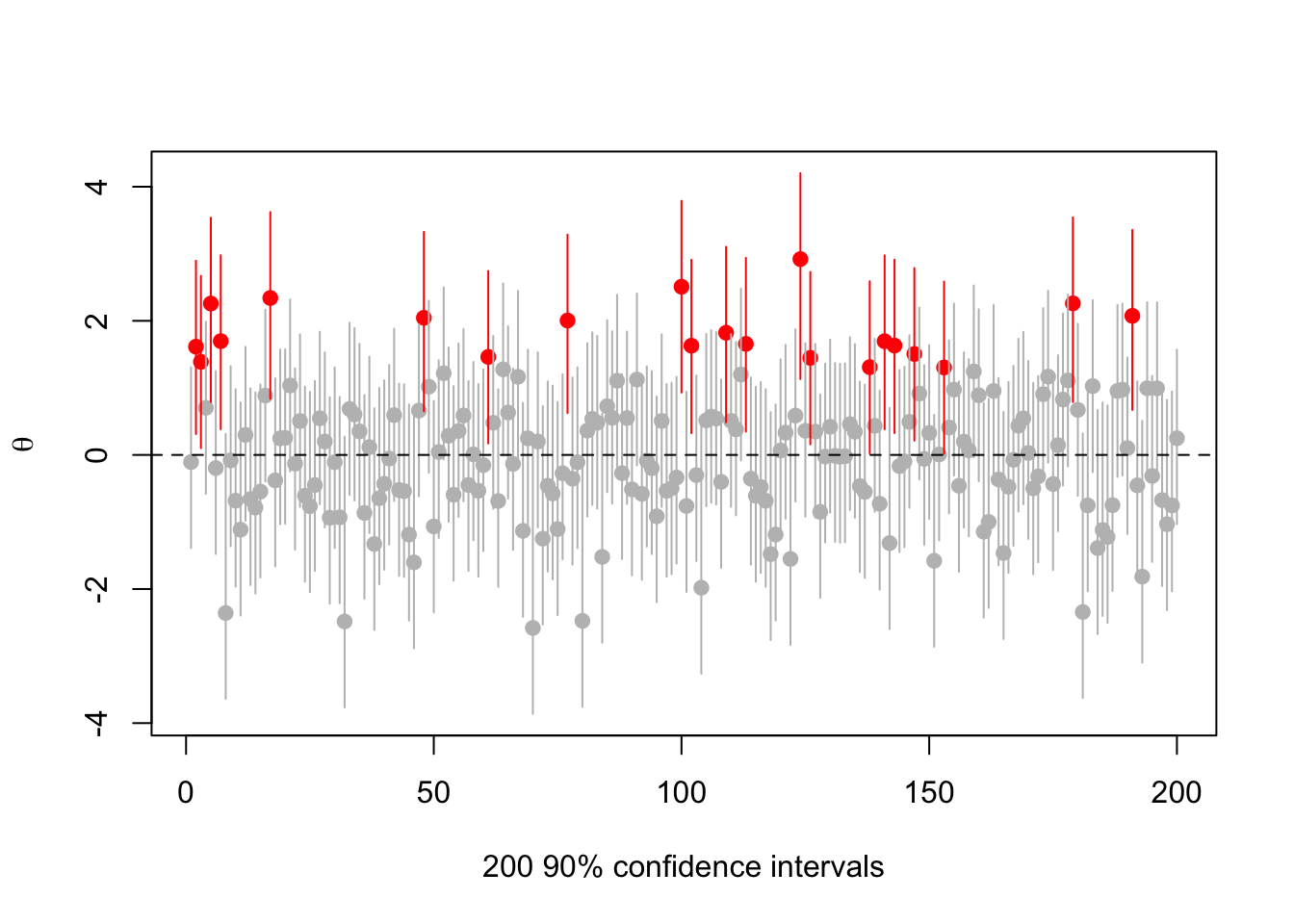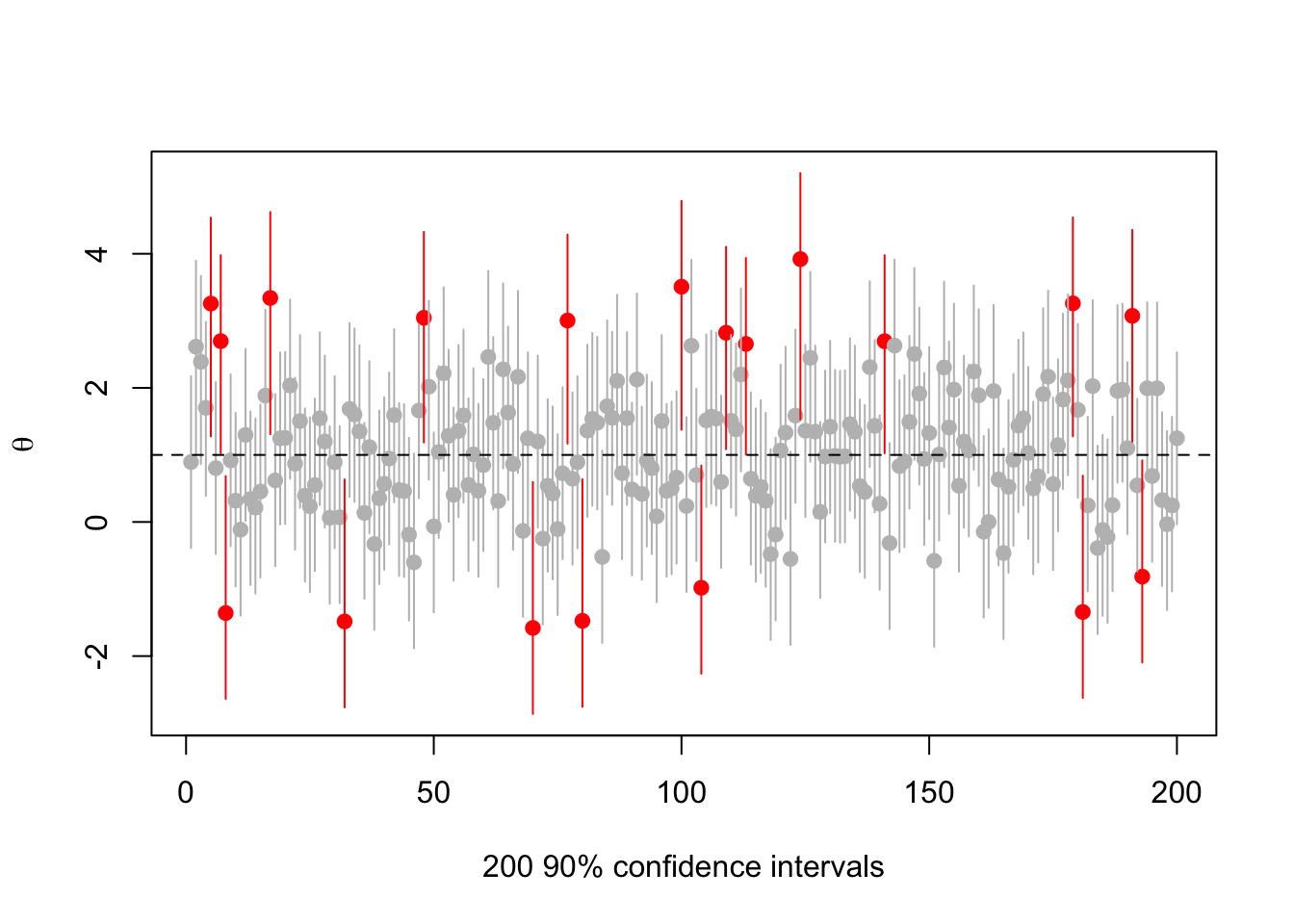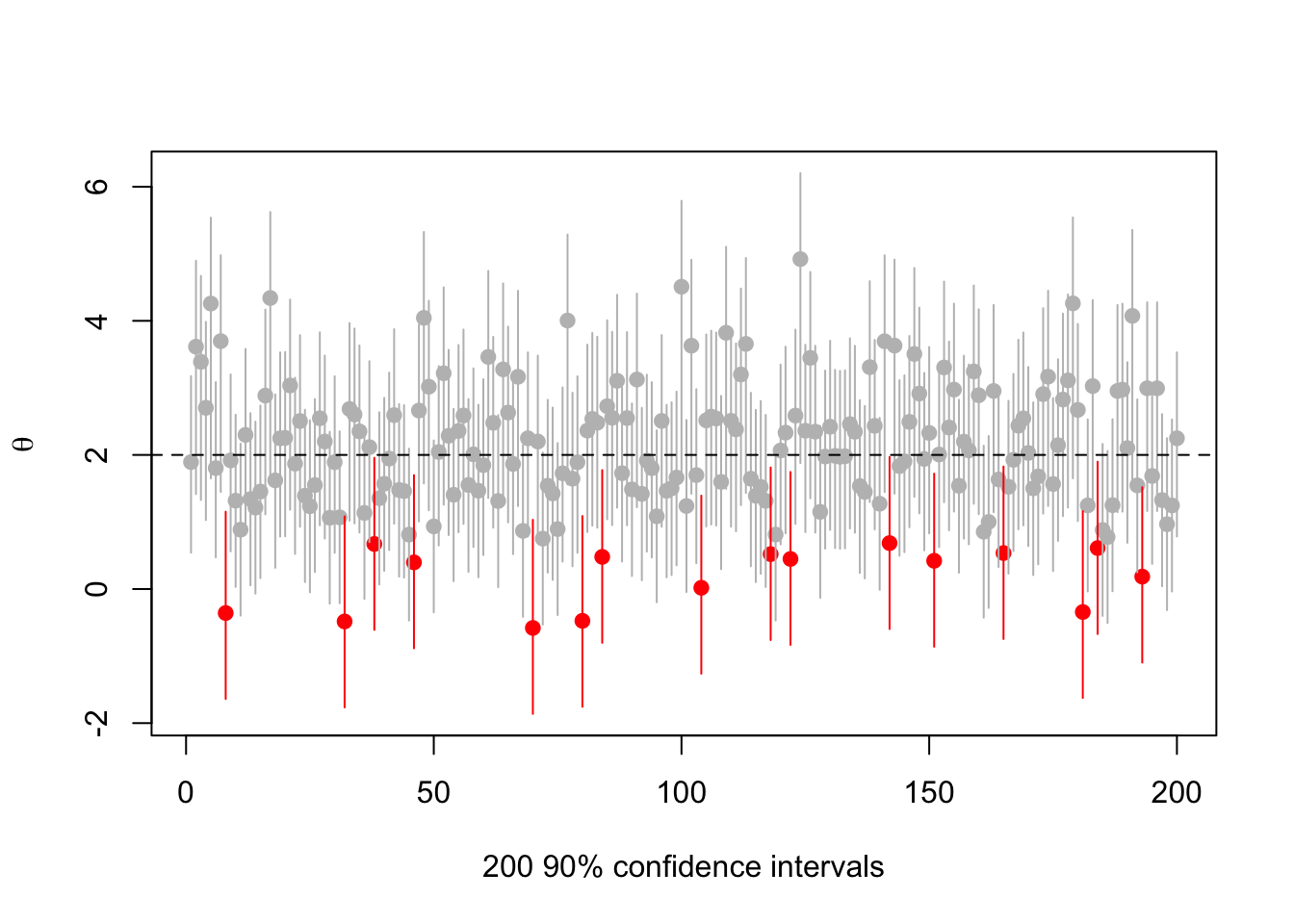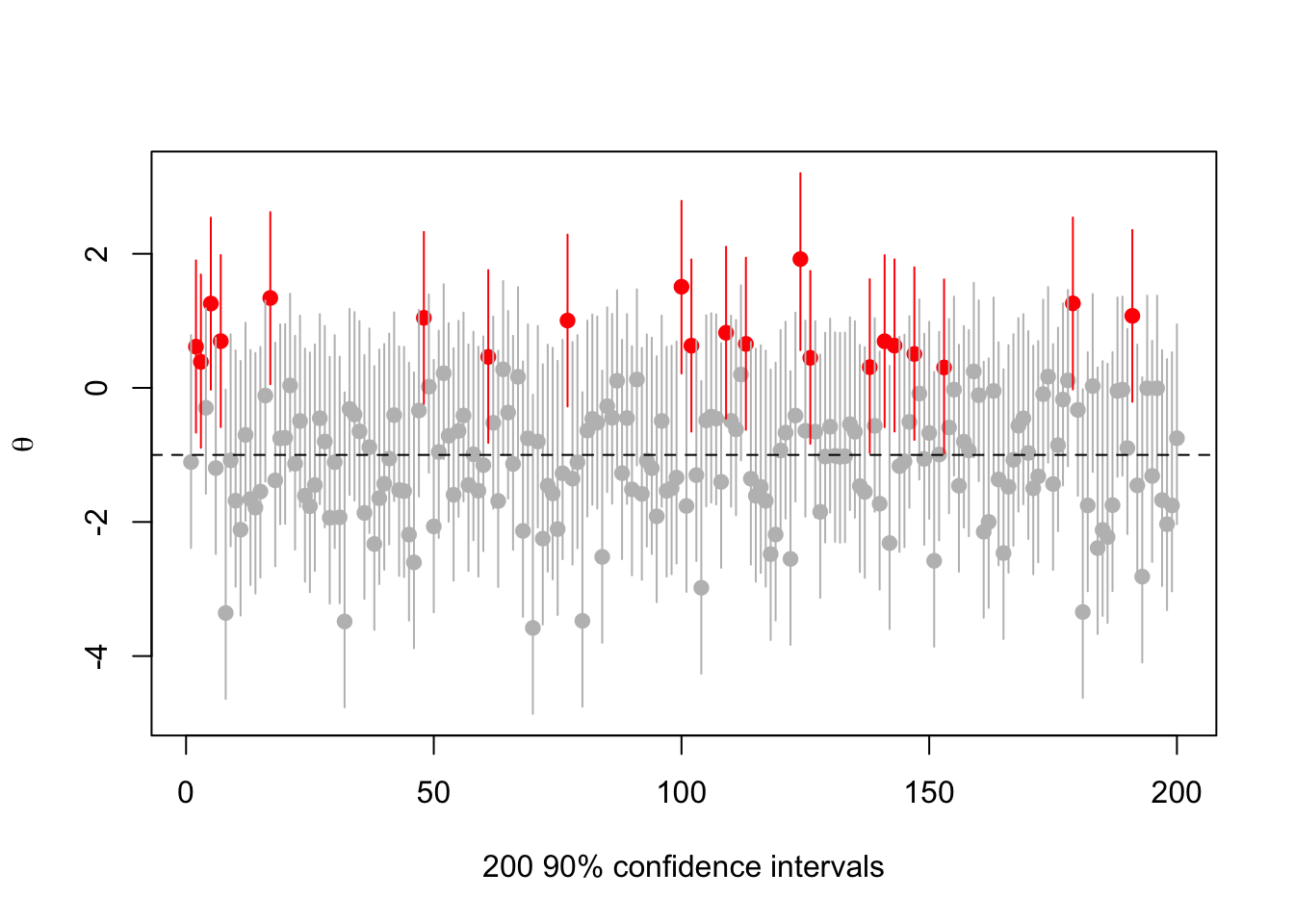# Confidence intervals: not a very strong property

It’s important for non-Bayesians (or non-exclusively-Bayesians) to remember that being a 95% confidence interval procedure is a fairly weak property. It’s not that confidence intervals are necessarily bad, but if they aren’t, it’s because of other requirements.

As an extreme case, consider the all-purpose data-free exact confidence interval procedure for any real quantity: roll a d20 and set the confidence interval to be the empty set if you roll 20, and otherwise to be $$\mathbb{R}$$. That’s a valid confidence interval procedure, it’s just a stupid one.

A much more interesting example comes from a recent paper by Kyle Burris and Peter Hoff, in the context of small-area sampling. First, suppose you have a random variable $$y\sim N(\theta, \sigma^2)$$ with $$\sigma^2$$ known. They say “it is easily verified that” for any function $$s:\mathbb{R}\to[0,1]$$, $C_s = \{\theta:y+\sigma z_{\alpha(1-s(\theta))} <\theta< y+\sigma z_{1-\alpha s(\theta)} \}$ defines a $$1-\alpha$$ confidence interval. If you stare at this for a while, you seee that you’re doing a level-$$\alpha$$ test at each value of $$\theta$$, but that $$s(\theta)$$ tells you how to split $$\alpha$$ between upper and lower tails. With $$s(\theta)=1/2$$ you get the standard equal-side test; with $$s(\theta)=0$$ you get the lower side only; with $$s(\theta)=1$$ you get the upper side only.

What’s unusual is that you get to choose $$s(\theta)$$ separately for each $$\theta$$. That’s fine from the formal definition of confidence intervals: the $$1-\alpha$$ coverage property only needs to hold for the true $$\theta$$, so we need only consider one $$\theta$$ at a time. But it’s a bit weird.

In the small-area sampling context you have lots of $$\theta$$s, $$y$$s, $$\sigma$$s, and so on, one for each small area, and you get sets of intervals $C^j_s = \{\theta:y_j+\sigma_j z_{\alpha(1-s_j(\theta))} <\theta< y_j+\sigma_j z_{1-\alpha s_j(\theta)} \}$

From a sampling/frequency point of view, the true $$\theta_j$$ are fixed, and $$y_j-\theta_j$$ depends only on sampling variability. So it’s perfectly ok to allow $$s_j(\theta)$$ to depend on data from areas other than area $$j$$.

In particular, you might have a prior for $$\theta_j$$ based on the data from all the other areas, say $$\theta_j\sim N(\mu_j,\tau^2_j)$$, and you might want to have shorter confidence intervals when $$\theta_j$$ is near $$\mu_j$$. You can do this by biasing the two-sided test to reject on the lower side for $$\theta_j <\mu_j$$ and on the upper side for $$\theta_j>\mu_j$$. Specifically, Burris & Hoff define $g(\omega)= \Phi^{-1}(\alpha\omega)-\Phi^{-1}(\alpha(1-\omega))$ and then $s_j(\theta)= g^{-1}(2\sigma_j(\theta-\mu_j)/tau^2_j)$ and show this all works, and something similar works in the more-plausible setting where you don’t know the $$\sigma$$s.

In R

make.s<-function(mu, sigma,tau2,alpha){
g<-make.g(alpha)
ginv<-make.ginv(alpha)

function(theta) ginv(2*sigma*(theta-mu)/tau2)
}

make.g<-function(alpha){
function(omega) qnorm(alpha*omega)-qnorm(alpha*(1-omega))
}
make.ginv<-function(alpha){
g<-function(omega)  qnorm(alpha*omega)-qnorm(alpha*(1-omega))
function(x){
f<-function(omega) g(omega)-x
if (x<0) {
uniroot(f,c(pnorm(x/alpha),0.5))$root } else if (x==0) { 0.5 } else uniroot(f,c(0.5,pnorm(x/alpha)))$root
}
}

ci<-function(y, sigma, s,alpha){

f_low<-function(theta) theta-(y+sigma*qnorm(alpha*(1-s(theta))))
f_up<-function(theta) (y+sigma*qnorm(1-alpha*s(theta)))-theta

low0<-y+qnorm(alpha/2)*sigma
low1<-low0
low<-if (f_low(low1)>0){
while(f_low(low1)>0) low1<- y+(low1-y)*2
uniroot(f_low,c(low1,low0))$root } else{ uniroot(f_low,c(low0,y))$root
}
hi0<-y+qnorm(1-alpha/2)*sigma
hi1<-hi0
high<-if (f_up(hi1)>0){
while(f_up(hi1)>0) hi1<- y+(hi1-y)*2
uniroot(f_up,c(hi0,hi1))$root } else{ uniroot(f_up,c(y,hi0))$root
}

c(low,high)
}

There’s a sense in which this has got to be cheating. In some handwavy sense, you’re sort of getting away with a $$1-2\alpha$$ confidence interval near $$\mu_j$$ at the expense of overcoverage elsewhere. But not really. The intervals really have $$1-\alpha$$ coverage: if you generated data for any true $$\theta$$ and used this procedure, $$1-\alpha$$ of the intervals would cover that true $$\theta$$, whatever it was. It’s all good.

But there’s nothing in the maths that requires $$\mu_j$$ to be a prior mean. It could also be that $$\mu_j$$ is the value you want to convince people that $$\theta_j$$ has. Again, you’ll get shorter confidence intervals when they’re centered near your preferred value and longer ones when they aren’t; again, they’ll have $$1-\alpha$$ coverage

Here’s an example with the true $$\theta=0$$, with $$\sigma=1$$, $$\mu=1$$, $$\tau=1$$, and with $$\alpha=0.1$$ to make it easier to see. So, we’d like to convince people that $$\theta\approx 1$$, but we aren’t allowed to mess with the 90% confidence level

set.seed(2019-6-11)
s<-make.s(1,1,1,.1)
rr<-replicate(200,{a<-rnorm(1);c(a,ci(a,1, s, 0.1))})
cover<-(rr[2,]<0) & (rr[3,]>0)
plot(1:200, rr[1,],ylim=range(rr),pch=19,col=ifelse(cover,"grey","red"),
ylab=expression(theta),xlab="200 90% confidence intervals")
segments(1:200,y0=rr[2,],y1=rr[3,],col=ifelse(cover,"grey","red"))
abline(h=0,lty=2)There are 21 intervals that miss zero, consistent with expectations. But they’re all centered at positive $$\theta$$.

What happens if we vary the true $$\theta$$? Try $$\theta=1$$ (now the red intervals are those that don’t cover 1)

set.seed(2019-6-11)
s<-make.s(1,1,1,.1)
rr<-replicate(200,{a<-rnorm(1,1);c(a,ci(a,1, s, 0.1))})
cover<-(rr[2,]<1) & (rr[3,]>1)
plot(1:200, rr[1,],ylim=range(rr),pch=19,col=ifelse(cover,"grey","red"),
ylab=expression(theta),xlab="200 90% confidence intervals")
segments(1:200,y0=rr[2,],y1=rr[3,],col=ifelse(cover,"grey","red"))
abline(h=1,lty=2)Or $$\theta=2$$

set.seed(2019-6-11)
s<-make.s(1,1,1,.1)
rr<-replicate(200,{a<-rnorm(1,2);c(a,ci(a,1, s, 0.1))})
cover<-(rr[2,]<2) & (rr[3,]>2)
plot(1:200, rr[1,],ylim=range(rr),pch=19,col=ifelse(cover,"grey","red"),
ylab=expression(theta),xlab="200 90% confidence intervals")
segments(1:200,y0=rr[2,],y1=rr[3,],col=ifelse(cover,"grey","red"))
abline(h=2,lty=2)Or $$\theta=-1$$

set.seed(2019-6-11)
s<-make.s(1,1,1,.1)
rr<-replicate(200,{a<-rnorm(1,-1);c(a,ci(a,1, s, 0.1))})
cover<-(rr[2,]< -1) & (rr[3,]> -1)
plot(1:200, rr[1,],ylim=range(rr),pch=19,col=ifelse(cover,"grey","red"),
ylab=expression(theta),xlab="200 90% confidence intervals")
segments(1:200,y0=rr[2,],y1=rr[3,],col=ifelse(cover,"grey","red"))
abline(h=-1,lty=2)There’s nothing actually wrong here, but it’s messing with people’s natural misunderstandings of confidence intervals in order to push the impression that $$\theta\approx 1$$.

I think I like it. Or maybe I don’t. One or the other.